Courses

# Test: Analysis of Stress & Strain Level - 2

## 20 Questions MCQ Test Engineering Mechanics | Test: Analysis of Stress & Strain Level - 2

Description
This mock test of Test: Analysis of Stress & Strain Level - 2 for Mechanical Engineering helps you for every Mechanical Engineering entrance exam. This contains 20 Multiple Choice Questions for Mechanical Engineering Test: Analysis of Stress & Strain Level - 2 (mcq) to study with solutions a complete question bank. The solved questions answers in this Test: Analysis of Stress & Strain Level - 2 quiz give you a good mix of easy questions and tough questions. Mechanical Engineering students definitely take this Test: Analysis of Stress & Strain Level - 2 exercise for a better result in the exam. You can find other Test: Analysis of Stress & Strain Level - 2 extra questions, long questions & short questions for Mechanical Engineering on EduRev as well by searching above.
QUESTION: 1

### The maximum shear due to an axial compression of 100 MPa is

Solution: Maximum shear stress,

τ =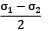=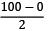τ = 50 MPa

QUESTION: 2

### When a rod of cross-sectional area 100 mm2is subjected to an tensile force 6kN, the maximum shear stress in the rod will be

Solution: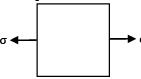σ =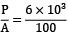= 60 MPa

τmax = σ/2 = 30 MPa

QUESTION: 3

### A body is subjected to two normal stresses 20 kN/m2 (tensile) and 10 kN/m2 (compressive) acting mutually perpendicular to each other. The maximum shear stress is

Solution: τmax =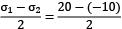= 15 kN/m2
QUESTION: 4

A body is subjected to a tensile stress of 1200 MPa on one plane and another tensile stress of 600 MPa on a plane at right angles to the former. If is also subjected to shear stress of 400 MPa on the same planes. The maximum shear stress will be

Solution: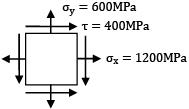maximum Shear Stress , τmax

τmax =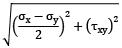=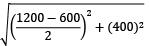= 500 MPa

QUESTION: 5

A Plane stressed element is subjected to the state of stress given by σx = τxy = 100 kgf/cm2 and σy = 0. Maximum shear stress in the element is equal to

Solution: Maximum shear stress,

τmax =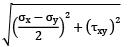=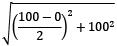= √12, 500

= 50√5 kgf/cm2

QUESTION: 6

The radius of Mohr’s circle for two unlike principal stresses of magnitude σ is

Solution: Radius of Mohr’s circle, τmax =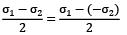τmax = σ

QUESTION: 7

At a point in a stressed material, the stress system is σx = + 800N/mm2 , σy = 0 and q = 0.

The radius of the Mohr’s stress circle for this case is__________ units.

Solution: σx = 800 N/mm2, σy = 0, q = τxy = 0

Radius of Mohr’s circle = τmax

τmax =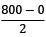=400 N/mm2

QUESTION: 8

The principal stresses at a point are 150.0 MPa and − 50.0 MPa; the radius of the corresponding Mohr’s circle will be

τmax =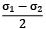=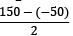= 100 MPa

QUESTION: 9

A spherical ball of volume 106 mm3 is subjected to a hydrostatic pressure of 90 MPa. If the bulk modulus for the material is 180 GPa, the change in the volume of the ball is

Solution: Volume = 106 mm3 Hydrostatic pressure, P = 90 MPa

Bulk modulus, k = 180 kN/m3 + 2

k =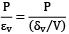180 × 103 =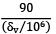δv = 500 mm3

QUESTION: 10

An element is subjected to px = 35 MPa, py = 20 MPa and shear stress q = 7.5 MPa. Then the direction of principal stresses is

Solution:

tan 2α =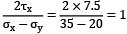tan 2α = 1 2α = 45°

α = 45°/2

= 22.5°

QUESTION: 11

For an inclined plane in a rectangular block subjected to two mutually perpendicular normal stresses 1000 MPa and 400 MPa and shear stress 400 MPa, the maximum normal stress will be

Solution: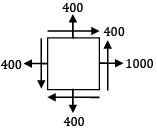σ1 =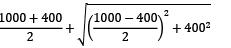= 700 + √9 × 104 + 16 × 104

= 700 + 500 = 1200MPa

QUESTION: 12

The normal stresses of 55 N/mm2 tensile and 45 N/mm2 compressive on two mutually perpendicular planes are acting at a point in a piece of elastic material. These planes also carry shear stress of 50 N/mm2. The minor principal stress will be

Solution: Minor principal stress, σ2

σ2 =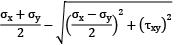=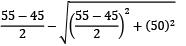σ2 = 65.71 N/mm2 compressive

σy = 45 N/mm2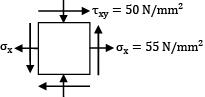QUESTION: 13

A rectangular strain rosette, shown in figure give following reading in a strain measurement task ϵP = 100 × 10−6, ϵQ = 150 × 10−6, ϵR = 200 × 10−6. The major principal strain is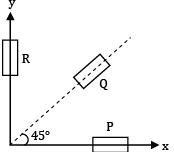Solution: ϵ1,2 =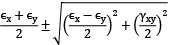ϵx = ϵP = 100 × 10−6

ϵy = ϵR = 200 × 10−6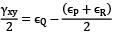= 150 × 10−6 − 150 × 10−6

= 0

ϵ1,2 =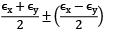ϵ1 =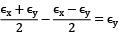ϵ2 =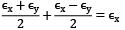ϵ1 = ϵy = 200 × 10−6

QUESTION: 14

If major and minor principal strains are given as 500 × 10−6 and − 200 × 10−6. Then calculate the maximum shear strain.

Solution: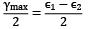⇒ γmax = (ϵ1 − ϵ2) = [500 − (−200)]10−6

= 700 × 10−6

QUESTION: 15

A rectangular strain rosette, shown in figure gives following reading in a strain measurement task ϵ1 = 1000 × 10−6, ϵ2 = 800 × 10−6 and ϵ3 = 600 × 10−6. The direction of the major principal strain with respect to gauge 1 is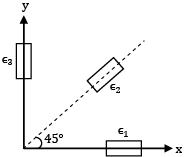Solution:

tan 2θp =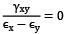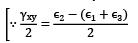=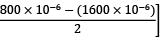= 0

QUESTION: 16

Consider the following failure criteria and match them with their corresponding graphical representations.

P - Maximum principal stress Theory

Q - Maximum distortion energy Theory

R - Maximum principal strain Theory

I.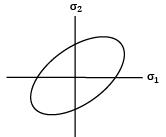II.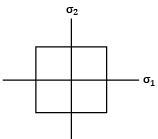III.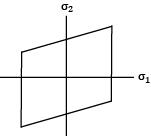Common Data for Question No: 17 and 18 If the principal stress at a point in an elastic material are 2σ, σ and − σ/2 the properties are Syt = 200 MPa and μ = 0.3

Solution:
QUESTION: 17

The value of σ at failure according to maximum principal stress theory

Solution: According to MPST

σ1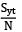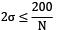[N = 1 at failure]

σ = 100 MPa

QUESTION: 18

The value of σ at failure according to strain energy theory

Solution: According to strain energy theory

σ12 + σ22 + σ32 − 2μ(σ1σ2 + σ2σ3 + σ3σ1) ≤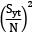4.95 σ2 = (200)2

σ = 89.89 MPa ≈ 90 MPa

QUESTION: 19

A spherical pressure shell 3.8 meters diameter and 3 mm thick is subjected to an internal pressure ‘P’. The elastic limit of the shell material in simple tension is 260 MPa. The factor of safety is to 2.8. The internal pressure, if the failure of shell is to be prevented, according to maximum shear stress theory is

Solution:

σnoop = σ1 = σ2 =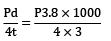Syt = 260 MPa

N = 2.8

According to MSST

Abs τmax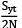Abs τmax =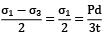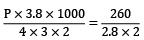P = 2.932 bar

QUESTION: 20

The force acting on a bolt consists of two components, an axial pull of 14 kN and a transverse shear load of 7 kN. The bolt material yield strength is 340 MPa and the factor of safety is 3 then, the cross sectional area of bolt required according to maximum shear stress theory is

Solution: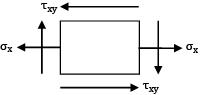σx =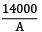τxy =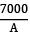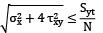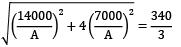A= 174.69 mm2

A ≈ 175 mm2